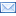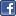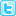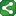## Sunday, March 8, 2009

### Displaying the Graphs (Bar charts) using PROC GCHART in SAS

Displaying the Graphs (Bar charts) using PROC GCHART in SAS :

Just a day ago, I've received a question on Graphs from one of the member of  my orkut community, which prompted me to create following example.

Below are 5 different types of Graphs were produced (including 3d graphs) using Proc Gchart.
Sample data set used for the examples:

data x;input quarter \$ ncl ram;
cards;
q1 20000 2000
q1 30000 3000
q1 45000 2000
q2 23000 2003
q2 45000 4500
q3 46000 5600
q3 89000 600
q3 67000 4500
q4 45890 890
q4 46000 9800

;
run;

*Example1: Simple barchart;

title 'Sales Report';

/* Produce the bar chart */
proc gchart data=x;vbar quarter / sumvar=ncl nozero ;
format ncl dollar8.;
run;
quit;
Snapshot of the output:

*Example2: Producing 3D graphs with cylinder shape using Proc Gchart;

pattern c=blue;
axis1 minor=none label=('NCL Sum');

title 'Bar Chart Shape';

/* use the SHAPE= option to specify a cylinder */

proc gchart data=x;
vbar3d quarter / sumvar=ncl discrete raxis=axis1 shape=cylinder
cframe=yellow autoref width=5;
format ncl dollar8.;
run;
quit;

Snapshot of the output:

axis1 minor=none label=('NCL Sum') offset=(0,0);

*Example3: Producing 3D graphs with reference lines using Proc Gchart;
/* use the FRONTFREF option to place reference lines in front of the bars */

proc gchart data=x;
vbar3d quarter / sumvar=ncl discrete raxis=axis1
coutline=black cframe=yellow width=
5vref=30 inside=sum frontref;
format ncl dollar8.;
run;
quit;

*Example4: Producing graphs after annotating the value above each midpoint bar using Proc Gchart;
*Create annotate dataset to assign the value on each midpoint bar;

data annotate;
length function color style text \$ 8;
retain function 'label' color 'black' when 'a' style 'swiss' xsys ysys '2' position '2' size 3 hsys '3' ;
set x;
by quarter;

/*calculating the cumulative totals for each quarter;*/
if first.quarter then final=ncl;
else if ^first.quarter then final+ncl;
midpoint=quarter;
if last.quarter then text=left(put(final,dollar8.));
y=ncl;
run;

/* Define colour patterns for bars */pattern1 c=blue;
pattern2 c=green;
pattern3 c=red;
pattern4 c=yellow;

Title 'Sales per Quarter Report';
/* Produce the bar chart */proc gchart data=x;
vbar quarter /discrete sumvar=ncl
/* subgroup – Width – width of the bar...... Space – space between two bars
Annotate – write the total value on the top of each bar.*/
subgroup=quarter
width=7space=2.0annotate=anno
;
format ncl dollar8.;
run;
quit;

Snap shot of the output:

5)Here is another way to create a graph..
I've added the titles and footnotes.... to this graph..

goptions reset=global gunit=pct border cback=white

colors=(black red green blue) ftitle=swissb
ftext=swiss htitle=6 htext=4

title1 'NCL vs Quarter';
footnote1 h=3 j=r 'Sarath';

axis1 label=none
origin=(24,);
axis2 label=none
minor=(number=1)
offset=(,0);
legend1 label=none
shape=bar(3,3)
cborder=black
cblock=gray
origin=(24,);

pattern1 color=lipk;
pattern2 color=cyan;
pattern3 color=lime;
pattern4 color=red;
pattern5 color=blue;

proc gchart data=x;
format ncl dollar8.;
vbar3d quarter / sumvar=ncl
subgroup=ncl
outside=sum
width=9
space=7
maxis=axis1
raxis=axis2
coutline=black
legend=legend1;
run;
quit;

Snapshot of the output:

SAS GRaphAnonymous said...

I like this website because its so informative. Thanks

Erick Furst said...

I tested your code for "Producing graphs after annotating the value above each midpoint bar using Proc Gchart" and the values didn't show up in the graph series. There is any restriction to any version?

Thanks,

sarath said...

There is no restriction regarding the SAS version Erick. I've checked the code and it works in SAS8 and 9.X versions. Please check your code one more time.

Thanks,
SarathShareThis